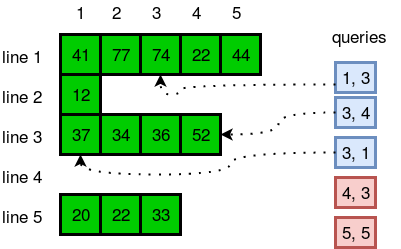## Algorithm

Problem Name: Java Arraylist

In this HackerRank Functions in Java programming problem solution,

Sometimes it's better to use dynamic size arrays. Java's Arraylist can provide you this feature. Try to solve this problem using Arraylist.

You are given n lines. In each line there are zero or more integers. You need to answer a few queries where you need to tell the number located in y**th position of x**th line.

Input Format
The first line has an integer n. In each of the next n lines there will be an integer d lines there will be an integer d space-separated integers. In the next line there will be an integer q denoting number of queries. Each query will consist of two integers x and y .

Constraints

• 1 <= n <= 20000
• 0 <= d <= 50000
• 1 <= q <= 1000
• 1 <= x <= n

Each number will fit in signed integer.
Total number of integers in n lines will not cross 10**5

Output Format
In each line, output the number located in y**th position of x**th line.If there is no such position, just print "ERROR!"

Sample Input

``````5
5 41 77 74 22 44
1 12
4 37 34 36 52
0
3 20 22 33
5
1 3
3 4
3 1
4 3
5 5
``````

Sample Output

``````74
52
37
ERROR!
ERROR!
``````

Explanation

The diagram below explains the queries:## Code Examples

### #1 Code Example with Java Programming

```Code - Java Programming```

``````
import java.io.*;
import java.util.*;
import java.text.*;
import java.math.*;
import java.util.regex.*;

public class Solution{
public static void main(String[] args){
Scanner scan = new Scanner(System.in);

int n = scan.nextInt();
ArrayList[] arr = new ArrayList[n];

for(int i = 0; i < n; i++){
int a = scan.nextInt();
arr[i] = new ArrayList();
for(int j = 0; j < a; j++){
int b = scan.nextInt();
}
}

int q = scan.nextInt();
for(int i = 0; i < q; i++){
int a = scan.nextInt();
int b = scan.nextInt();
try{
System.out.println(arr[a - 1].get(b - 1));
}catch(Exception e){
System.out.println("ERROR!");
}
}
}
}
``````
Copy The Code &